# mysql 实现select 判断查询

mysql查询A表type字段等于1的num字段合计值和type字段等于2的num字段合计值,

sql语句:

``````select sum(if(type=1,num,0)) as total_1,sum(if(type=2,num,0)) as total_2 from a ;
``````

sql语句:

``````select sum(CASE WHEN type=1 THEN num ELSE 0 END) as total_1,sum(CASE WHEN type=2 THEN num ELSE 0 END) as total_2 from a ;
``````

``````select sum(CASE type WHEN 1 THEN num ELSE 0 END) as total_1,sum(CASE type WHEN 2 THEN num ELSE 0 END) as total_2 from a ;
``````

##### 热门推荐
•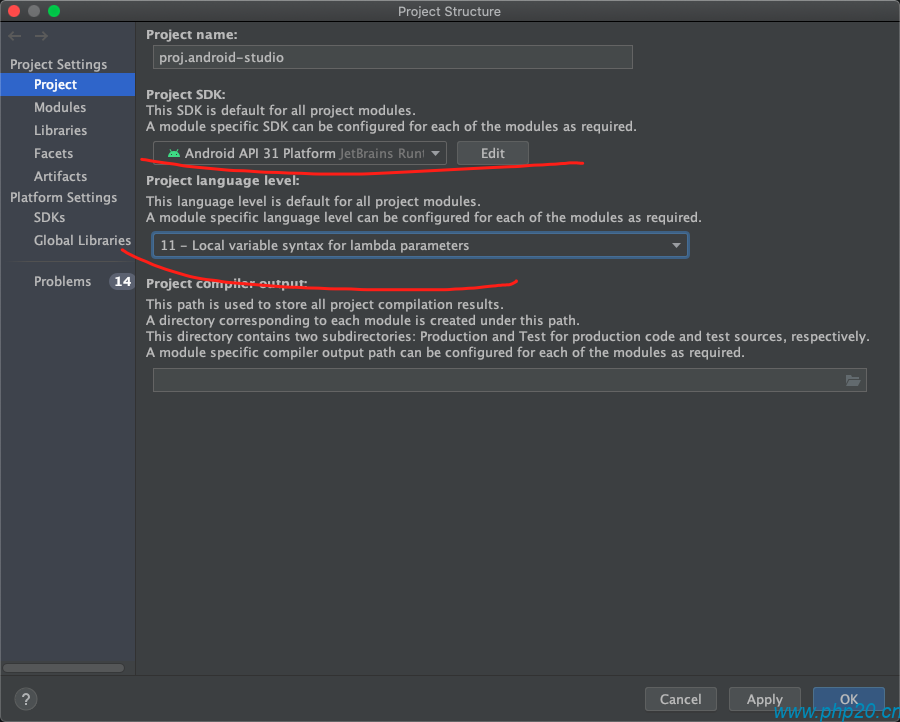浏览(1,986)
•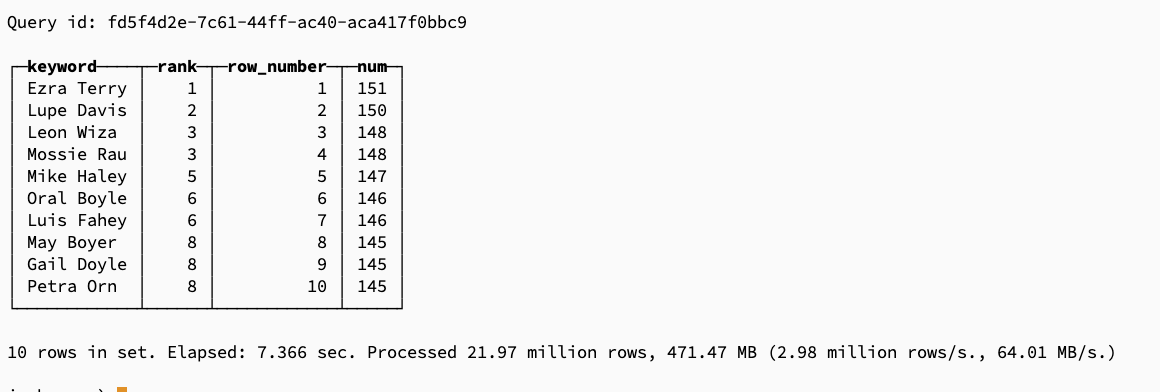浏览(791)
•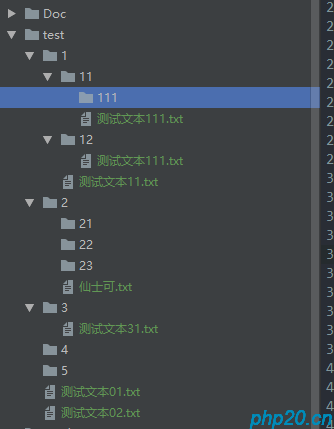浏览(782)
••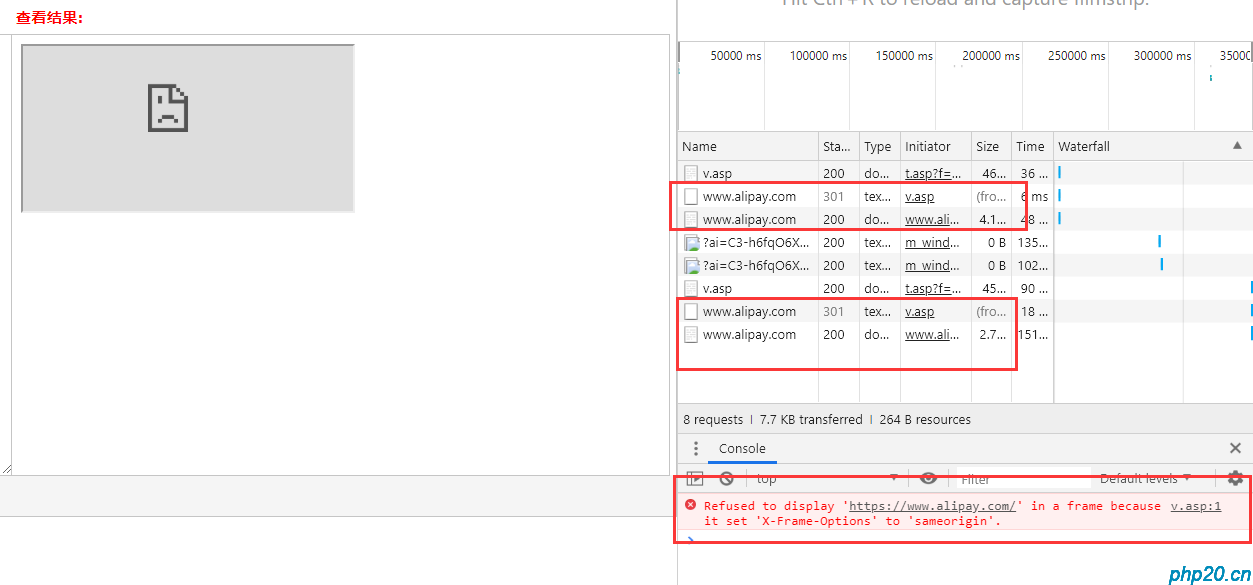浏览(685)
•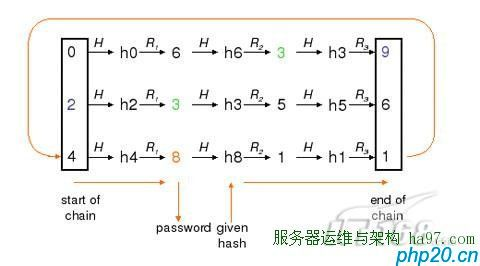•浏览(641)
•浏览(539)
•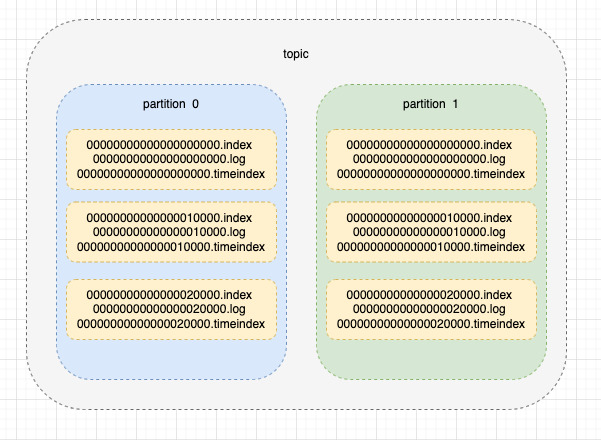浏览(487)
•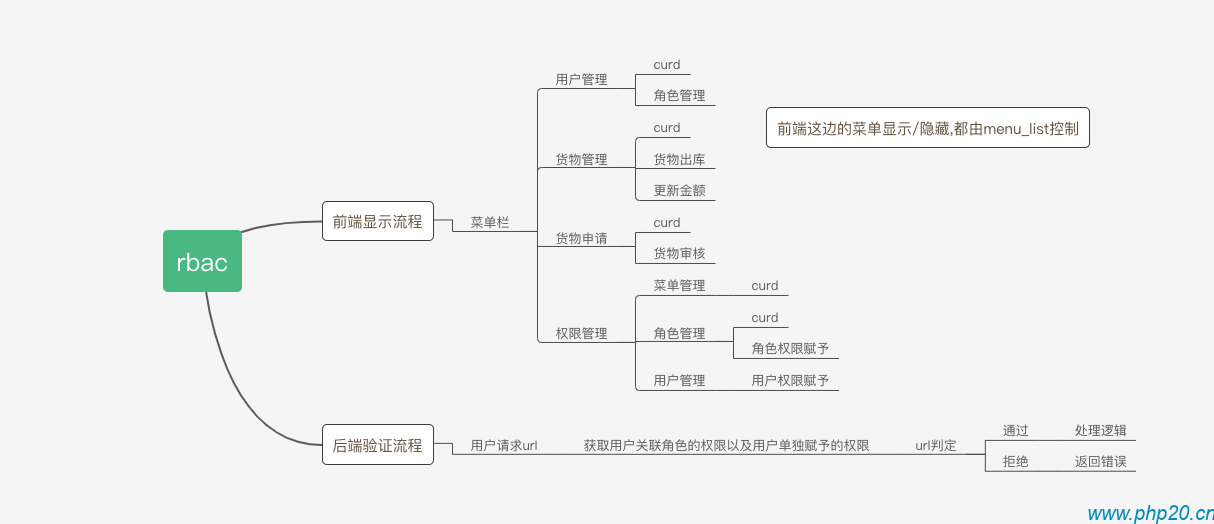浏览(477)